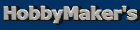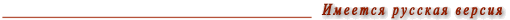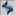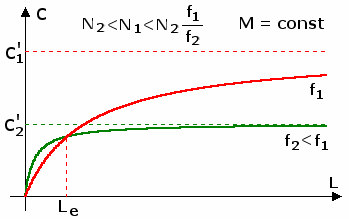Main pageList of articles
 Blurring the Background A scientific approach ______________________________________________ A wish to have some fuzziness on a photograph is quite natural. First, the human eye sees in a similar way. Second, this can make shots artistically expressive. This article deals with some physical peculiarities that determine the degree of background fuzziness produced by photographic lenses. It is important to understand that only the degree of fuzziness will be discussed. Any subtle details, such as bokeh etc., are beyond the scope of this article. With this in mind, let us recall the formula that describes the sharpness area behind the object: L behind = (c N ) / (M2 (1 - (d / h))), where h - hyperfocal length (h = f2 / (c N) ); f - focal length; N - f-number (or f-stop) (1,4; 2; 2,8; 4, 5,6; 8; ģ) c - diameter of largest acceptable circle of confusion; d - object distance; M - magnification (M = f / d). Do you see this formula for the first time in your life? Confused over the circle of confusion? Any other questions? Then consider reading a good book on optics. As a last resort, you may also read my text entitled "Depth of Field: A combination of unknown and obvious facts". Let us rewrite the formula to solve for the circle of confusion: c = (M2 f L) / (N (f + L M)). Now it shows the diameter of an image circle produced by a background point, L being the distance between the object and the background. The formula is approximate. It holds under the same assumptions as for the initial one. Some transformations yield: c = c' (1 / (1 + (f / (M L)))), where c' = (Mf) / N or c = c' (1 / (1 + (d / L))) Let us discuss the result we derived. The most important conclusions are as follows. 1. The first conclusion is quite obvious. The farther the background, the larger degree of its fuzziness. However, the infinitely far background is not infinitely blurred. Points at infinity are rendered as circles with the diameter c' = (Mf) / N. Closer points are blurred to a lesser degree. If magnification is constant, the fuzziness of infinity is proportional to the focal length. If we keep the distance to the object constant and shoot at various magnifications, c' is proportional to the square of the focal length. 2. The background fuzziness is always inversely proportional to N. This means that a one-step change in f-stop causes the fuzziness to change by 1.4 times. 3. Let us assume we have two lenses with different focal lengths. The distance between the object and the background is constant. What f-stops should we set for each lens to obtain the same degree of fuzziness? The following formula gives the answer: N2 / N1 = (f2 / f1) ((f1 + M L)/(f2 + M L)) . If the background is infinitely far, it simplifies: N2 / N1 = (f2 / f1). Let us consider a couple of illustrations. Example 1: Magnification equals 0.05. What f-stop should we choose for the 135 mm lens to blur the infinitely far background to the same degree as for the 50 mm lens at an f-stop of 4? The answer to this is 11 (10.8). Example 2: Let us assume that the distance between the object and the background is 1 m. Now, for the same lenses and f-stops, we should set the 135 mm lens to an f-stop of 5.6 (5.8). 4. Let us consider another example. Suppose, we have decided to make a portrait and shoot it at a magnification of 0.05. We are going to use two lenses: a 135 mm lens @ f/8 and a 50 mm lens @ f/4.5. First of all, note that the depth of field will be two times larger for the first lens. However, despite this larger depth of field, it will blur the infinitely distant background to a greater extent (c' = 0.84 mm for the 135 mm lens, while it is only 0.56 mm for the 50 mm lens). The results are not surprising. Now let us consider the background that is 1 m away from the object. At first, the result may astonish you. The 50 mm lens blurs the close background to a greater extent. We have the circle of confusion of 0.23 mm for the first lens, and 0.28 mm for the second. But that is true indeed! If we have two lenses and f2 < f1 and N2 < N1 < ((N2 f1)/f2), then there is a certain distance at which both of the lenses equally blur the background. This distance is determined by the formula: Le = (f1 f2 (N1 - N2)) / (M (N2 f1 - N1 f2) Under such conditions, close backgrounds are blurred more intensely by lenses with relatively short focal lengths, while distant backgrounds are blurred to a greater extent by lenses with long focal lengths. (See also the picture below.)5. How far should a background be to be considered as infinitely distant? In other words, where does the infinity start from? From our main formula it is easy to derive that if L = 9 d, then the background fuzziness is 90 per cent of c'. Note that this estimate depends neither on N, neither on f. Thus, for any given lens infinity starts from 10d, if measured from the camera. Of course, it is not the absolute criterion. If you are unhappy with it, develop your own estimation. *** But the main conclusion remains as follows: one has to shoot more and to gain experience to understand how the formulas work. Most photographers have never seen and used the above formulas. Nevertheless, they can create truly wonderful pictures. However, if math does not get you sick and tired, you may play with a simple calculator based on an MS Excel file. Feel free to customize it to your own needs. Download Blur_02.xls.Main pageList of articles ® Igor Yefremov, 2002, all rights reserved You must obtain a written permission from me to use any materials of this site for any commercial or non-commercial purposes, unless there is an explicit statement to the contrary.
ą░ą▓čéąŠą╝ą░čéčŗ ąŠąĮą╗ą░ą╣ąĮ http://www.avtomaty-besplatno.com ą┐ąŠąĖą│čĆą░čéčī ą▒ąĄčüą┐ą╗ą░čéąĮąŠ